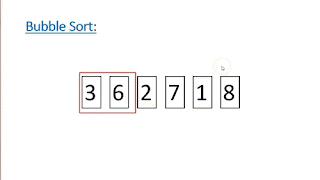## Labels

Linux (28) Python (13) Raspberry Pi (5) Bugs (3) Install (3) C (2) Brainf**k (1) HTML (1) Maths (1) Sorts (1)

## Monday, 12 November 2018

### Bubble sort in Python vs C

Sorting algorithms are used to put a elements of a list into a certain order. One example of a sorting algorithm is the bubble sort. The bubble sort works by comparing successive pairs of numbers in the list and then stops when no more swaps are required. The bubble sort is not a very efficient sorting algorithm.Firstly lets write a bubble sort in Python that sorts the numbers into ascending order.

Create an array of filled with random numbers:
`numbers=[3,5,2,8,6,13,4,1,44]`

Worst case scenario we have to loop through all the numbers once for each number in the list:
```arrayLen=len(numbers)
for i in range(0, arrayLen):
```
Each time we loop through all the numbers the biggest number is moved to the end so we do not need to compare this value again as we already know that is the biggest. We also do not need to go to the last element as we compare it to the next element in the list.
```for j in range(0, arrayLen-i-1):
if( numbers[j] > numbers[j+1] ):
numbers[j], numbers[j+1] = numbers[j+1], numbers[j]
```

So putting it all together:
```numbers=[3,5,2,8,6,13,4,1,44]
for i in range(0, arrayLen):
for j in range(0, arrayLen-i-1):
if( numbers[j] > numbers[j+1] ):
numbers[j], numbers[j+1] = numbers[j+1], numbers[j]
```

But this code will carry on even when the numbers are already in order so we need to add a if statement to stop the program when no more swaps are being made.

```numbers=[3,5,2,8,6,55,4,1,44]
for i in range(0, len(numbers)):
swap=False
for j in range(0,len(numbers)-i-1):
if ( numbers[j] > numbers[j+1] ):
numbers[j], numbers[j+1] = numbers[j+1], numbers[j]
swap=True
if( swap == False ):
break
print(numbers)
```

This program now stops when no more swaps are being made and prints out the array after every pass.

The gives the result of :
```[3, 2, 5, 6, 8, 4, 1, 44, 55]
[2, 3, 5, 6, 4, 1, 8, 44, 55]
[2, 3, 5, 4, 1, 6, 8, 44, 55]
[2, 3, 4, 1, 5, 6, 8, 44, 55]
[2, 3, 1, 4, 5, 6, 8, 44, 55]
[2, 1, 3, 4, 5, 6, 8, 44, 55]
[1, 2, 3, 4, 5, 6, 8, 44, 55]
```

Now lets create an array of random numbers so we are not just testing the same numbers each time.
Change
```numbers=[3,5,2,8,6,55,4,1,44]
```
To
```numbers=[random.randint(1,10000) for _ in range(5000)]
```
And we will also need to import the random library by adding this line to the start of the program.
```import random
```

Lets now add a timer into the program so we can compare it to the C program.
Firstly import the time module by adding this line to the start
```import time
```
And add timing code so the final program looks like this
```import random
import time
numbers = [random.randint(1,10000) for _ in range(5000)]
start=time.time()
for i in range(0, 5000):
swap=False
for j in range(0,5000-i-1):
if ( numbers[j] > numbers[j+1] ):
numbers[j], numbers[j+1] = numbers[j+1], numbers[j]
swap=True
if( swap == False ):
break
end=time.time()
print(end-start)
```

Running this gives me a output of around 5s to 6s to sort a list of 5000 elements.

Now lets write a bubble sort in C using the same steps.

```#include <stdio.h>
#include <stdlib.h>
#include <time.h>
int main()
{
clock_t start,end;
double time_spent;
int numbers,temp,stop;
srand(time(0));
//Initalize array with 5000 random numbers
for(int z=0;z<5000;z++)
numbers[z]=rand()%10000;
//Start timer
start = clock();
for(int i=0; i < 5000; i++)
{
stop=0;
for(int j=0; j < 5000-1;j++)
{
//Swap numbers if element j is bigger than element j+1
if(numbers[j] > numbers[j+1]){
temp=numbers[j];
numbers[j] = numbers[j+1];
numbers[j+1] = temp;
stop=1;

}
}
//Stop if numbers are in order(no swaps occured)
if(!stop)
break;

}
//Stop timer
end = clock();
time_spent = (double)(end-start) / CLOCKS_PER_SEC;
printf("%lf\n", time_spent);
return 0;
}

```

Running this usually takes around 0.1s-0.2s which is a great improvement on Pythons 5s-6s. Although the test isn't perfect it shows how Python is much slower than C. On the other hand the Python script is much shorter, so we trade performance for development time.